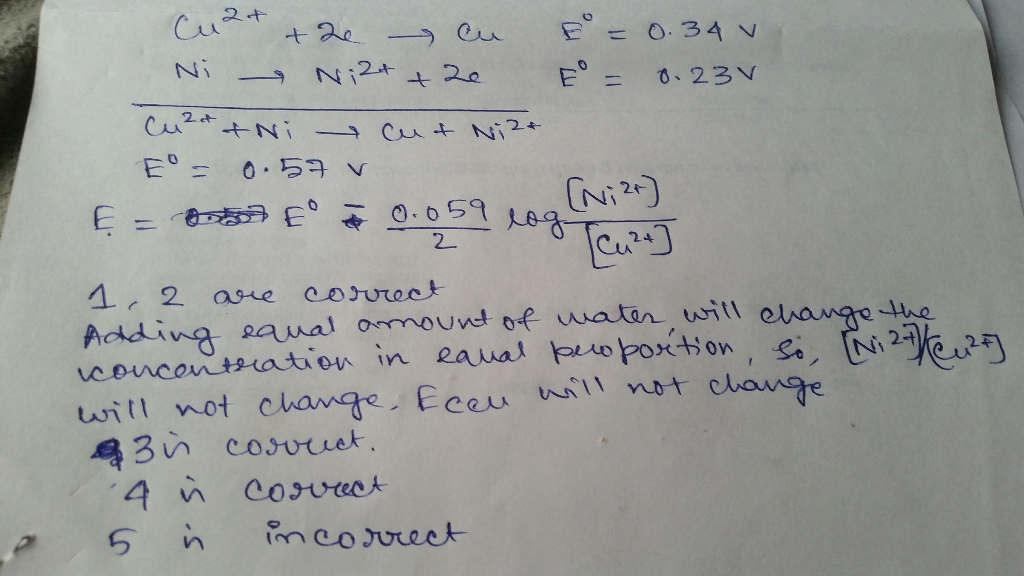# Question & Answer: Consider a cell based on the following line notation at 298 K: Cu | Cu2+ (0.289 M)…..

Consider a cell based on the following line notation at 298 K:

Cu | Cu2+ (0.289 M) || Ni2+ (1.25 M) | Ni

Don't use plagiarized sources. Get Your Custom Essay on
Question & Answer: Consider a cell based on the following line notation at 298 K: Cu | Cu2+ (0.289 M)…..
GET AN ESSAY WRITTEN FOR YOU FROM AS LOW AS \$13/PAGE

Given:

Cu2+ + 2 e → Cu     Eo = 0.34 V
Ni2+ + 2 e → Ni     Eo = -0.23 V

How many of the following responses are true?

1. Using a Pt electrode in place of the Cu electrode will not change the potential of the cell
2. Reduction takes place at the cathode
3. Adding equal amounts of water to both half reactions will not change the potential of the cell
4. Increasing the concentration of the Ni2+ will decrease the potential of the cell
5. Increasing the concentration of the Cu2+ will decrease the concentration of the reaction